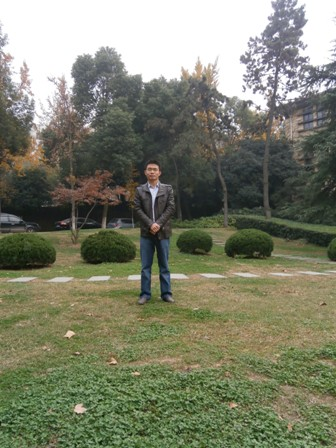# 刘学坤【简  介】

【开设课程

【主要论著

1中国共产党的团结思想探析[J].河海大学学报(哲学社会科学版).2018(03).

2论马克思恩格斯的共享观[J].江苏社会科学.2017(05).

3西方友爱伦理的观念史考察[J].当代中国价值观研究.2017(02).

4爱情与青年的公民友爱教育[J].青少年学刊.2017(02).

5组织视角的农村思想政治工作的现代性问题及对策[J].内蒙古农业大学学报(社会科学版).2016(04).

6水利特色高校德育在水利事业中的作用——以河海大学为例[J].华北水利水电大学学报(社会科学版).2016(01).（第一作者）

7改革情境中的思想政治教育活动方式的现代转型[J].甘肃理论学刊.2015(01).

8论思想政治教育实践的发展趋势[J].党政研究.2015(06).

9思想政治工作视角下的农民精神困境及对策[J].河海大学学报(哲学社会科学版).2013(02).

10学校教育与身体的尊严[J].教育理论与实践.2013(01).

11现代性视角的爱国主义及其教育再思考[J].广州大学学报(社会科学版).2012(09).

12康德的道德教育思想[J].南京邮电大学学报(社会科学版).2012(04).

13“科技下乡”的现代性语境及其教育功用:功能主义的解释[J].求实.2012(08).

14西方政治与伦理的分离以及政治教育的产生[J].高教发展与评估.2012(05).

15村落中的法律动员:农村普法的现代性语境和困境[J].三峡大学学报(人文社会科学版).2012(05).

16台湾地区大学教师专业伦理建设现状与启示[J].现代大学教育.2012(05).

17村落中的公民成长:社会转型期农民政治社会化现状与途径创新[J].思想政治教育研究.2012(02).

【主要科研项目

【荣誉奖励